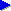# Hash tables (dictionaries)In this lecture, Prof Jonathan Shewchuk explains the technique of hashing, and the hash table structure. Hash tables are a data structure used to implement a "lookup table" that has on average constant access time, whatever the number of key/value pairs in the table.

Related pages on this site:Introduction to HashMapsHow hash functions workStatistical properties of hash codesJava hash map performance

## Lecture overview

TimeNotes / content / relevant pages on this site
0'00Introduction: notion of keys and hash codes
9'40Definition of terms: buckets and compression function
13'50Collisions (see the related page on hash code statistics)
16'50Chaining, the technique in fact used by Java hash map implementations (note that a technique not mentioned in this lecture is the possibility of storing just the hash code, rather than hash code and key pair, if the hash function is strong enough). See also: Introduction to hasing and hash maps, where we illustrate the notion of chained buckets.
19'40Diagram of a hash table structure
22'20Hash table operations
32'20Performance of hash tables
38'40Hash codes and compression functions. Note that the technique advocated here of using a prime modulo as the compression function is not actually the technique used by Java. Essentially for performance gain, Java's implementation ANDs the hash code with (buckets-1), always having a power of 2 number of buckets. As explained in our discussion of how hash functions work, a good hash function is one with a "random distribution", and normally, ANDing off the bottom bits of the hash code is risky if those bits of the hash codes exhibit poor randomness. To get round this problem, Java uses a supplementary hash function to "even out" random elements in the hash code across more of the bit range.